### IMO Shortlist 2007 problem C1

Kvaliteta:
Avg: 0,0
Težina:
Avg: 6,0
Let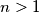$n > 1$ be an integer. Find all sequences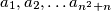$a_1, a_2, \ldots a_{n^2 + n}$ satisfying the following conditions: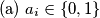$\text{ (a) } a_i \in \left\{0,1\right\}$ for all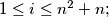$1 \leq i \leq n^2 + n;$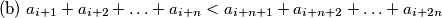$\text{ (b) } a_{i + 1} + a_{i + 2} + \ldots + a_{i + n} < a_{i + n + 1} + a_{i + n + 2} + \ldots + a_{i + 2n}$ for all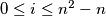$0 \leq i \leq n^2 - n$
Author: unknown author, Serbia
Izvor: Međunarodna matematička olimpijada, shortlist 2007